• C++调用matlab函数（未安装 matlab 也可以使用）
2022-05-21 18:54:59

在正常工作环境中往往是可以使用无线网络的，此时安装一个matlab也不是什么难事；但是也难免也会遇到一些工作电脑不允许链接无线网络，此时若安装一个matlab则是一件非常痛苦的事，因为其安装包就20多个G，当时我是用手机开热点下载的，仅仅下载安装包就浪费了一个下午+一个晚上；

下面就举一个例子，针对安装过matlab未安装过matlab的情况去介绍C++调用matlab函数的操作流程：

# 一、封装 matlab 函数

首先把 matlab 代码封装成函数的形式，下面举一个简单的函数为例；

function c = myadd(a, b)
c  = a + b;
end


# 二、编译 matlab 函数

具体的编译步骤如下：

### 2、输入 mbuild –setup 回车，然后点击选择 mex -setup C++ -client MBUILD；

（1）-W 是控制编译之后的封装格式；

（2）cpplib 是指编译成 C++ 的 lib，如果需要编译成 C 的 lib，就用 lib 即可，去掉 cpp；

（5）其他具体含义可以通过mcc –help命令查看，注意参数的大小写；

# 三、C++环境配置

说明：创建工程文件，注意：测试环境使用的是64位操作系统，因此务必在x64环境下进行以下配置。（这是与你的matlab版本匹配的，我的matlab安装的是64bit的）

环境配置步骤如下：

### 1、VC++目录

找到自己 Matlab 的安装目录按照以下进行配置；

（1）包含目录：D:\Matlab2020a\extern\include

（2）库目录：D:\Matlab2020a\extern\lib\win64\microsoft

说明：如果未安装matlab，则需要去已安装过 matlab 的电脑上找到上述两个路径所对应的文件

然后将其复制到该电脑，最后将其路径添加至包含目录和库目录即可！！！

### 3、配置系统变量（已修改）

“我的电脑”右键，打开“属性”；选择“高级系统设置”；找到系统属性页面的“高级”选项，右下方

“环境变量”；页面中下方是系统变量，将如下路径添加到环境变量的Path中：

（1）如果电脑上装的有 matlab 则仅需要添加如下路径：

D:\Matlab2020a\bin\win64

（2）如果电脑上没有安装 matlab 则需要添加如下路径：

D:\Matlab2020a\runtime\win64

D:\Matlab2020a\bin

D:\Matlab2020a\polyspace\bin

D:\Matlab2020a\bin\win64

说明：D:\Matlab2020a\runtime\win64 、D:\Matlab2020a\bin、D:\Matlab2020a\polyspace\bin

这三个路径在安装 matlab 时系统会自动将其添加到环境变量中，所以如果电脑上未安装 matlab

则需要去已安装过 matlab 的电脑上找到上述四个路径所对应的文件，然后将其复制到该电脑，最

后将其路径添加至环境变量即可！！！

注意：配置完环境变量后需要重启电脑，环境变量才会生效；

### 4、重新打开VS2015的项目，将Matlab生成的四个文件放入该项目所在文件夹下（已修改）

注：Project1 为创建的 C++ 工程；

# 四、代码测试

#include "addtest.h"

int main()
{
bool isOk = 0;
if (!mclInitializeApplication(NULL, 0))
{
std::cout << "Could not initialize the application.\n";
return -1;
}
std::cout << "isOk = " << isOk << std::endl;// isOK = 0
std::cout << "isOk = " << isOk << std::endl; // isOK = 1

mwArray a(1, 1, mxDOUBLE_CLASS);
mwArray b(1, 1, mxDOUBLE_CLASS);
a(1, 1) = 1.8;
b(1, 1) = 2.9;
mwArray z(1, 1, mxDOUBLE_CLASS);

std::cout << a << "+" << b << "=" << z << std::endl;

mclTerminateApplication();

system("pause");
return 0;
}



注意：在 c++ 中调用 matlab 需要处理 mwArray 接口问题；

具体处理办法见：mwArray和Mat之间的转化_一米九零小胖子的博客-CSDN博客

参考博客：https://blog.csdn.net/u011913417/article/details/102679274

该博客意在记笔记，纯属为了以后使用方便！！！矩阵 开发语言
更多相关内容
• 主要为大家详细介绍了C++调用Matlab函数求特征值，具有一定的参考价值，感兴趣的小伙伴们可以参考一下
• c++调用matlab函数，c++程序有很多种方法调用Matlab中的函数，但是我最喜欢的方法是直接调用Matlab的动态库，这样生成的程序在没有安装Matlab的机子上也可以运行。
• 之前已经跟大家介绍了在ubuntu系统下C++调用matlab程序的方法，需要的朋友们可以参考这篇文章，本文将给大家介绍关于windows下C++调用matlab程序的相关内容，分享出来供大家参考学习，下面话不多说了，来一起看看...
• C++ 调用MATLAB函数 调用带单一返回参数的函数 使用名称/值参数调用函数 以异步方式调用函数 使用多个返回参数调用函数 用原生 C++ 类型调用函数 控制输出的数目 另请参阅 从 C++ 调用MATLAB函数 使用...

目录

从 C++ 调用 MATLAB 函数

调用带单一返回参数的函数

使用名称/值参数调用函数

以异步方式调用函数

使用多个返回参数调用函数

用原生 C++ 类型调用函数

控制输出的数目

## 从 C++ 调用 MATLAB 函数

​        使用 matlab::engine::MATLABEngine 类的 feval 和 fevalAsync 成员函数从 C++ 调用 MATLAB® 函数。当要将函数参数从 C++ 传递给 MATLAB 和将函数执行的结果返回给 C++ 时，请使用这些函数。这些成员函数的工作方式类似于 MATLAB feval 函数。

​        要调用 MATLAB 函数，需要满足以下条件：

• 将函数名称作为 matlab::engine::String 传递。

• ​定义 MATLAB 函数所需的输入参数。可以使用原生 C++ 数据类型或 MATLAB 数据 API。有关详细信息，可以参考MATLAB 数据 API。​

• ​指定 MATLAB 函数应提供的输出的数目。默认为一个输出。有关详细信息，可以参考使用多个返回参数调用函数和控制输出的数目。​

• 为 MATLAB 函数的结果定义适当的返回类型。

• 使用流缓冲区将标准输出和标准错误从 MATLAB 命令行窗口重定向到 C++。有关详细信息，可以参考Redirect MATLAB Command Window Output to C++​

### 调用带单一返回参数的函数

此示例使用 MATLAB gcd函数求两个数值的最大公约数。MATLABEngine::feval 成员函数返回 gcd 函数调用的结果。使用 matlab::data::ArrayFactory 创建两个标量 int16_t 参数。将参数以 std::vector 形式传递给 MATLABEngine::feval。

#include "MatlabEngine.hpp"
#include "MatlabDataArray.hpp"
#include <iostream>
void callFevalgcd() {

// Pass vector containing MATLAB data array scalar
using namespace matlab::engine;

// Start MATLAB engine synchronously
std::unique_ptr<MATLABEngine> matlabPtr = startMATLAB();

// Create MATLAB data array factory
matlab::data::ArrayFactory factory;

// Pass vector containing 2 scalar args in vector
std::vector<matlab::data::Array> args({
factory.createScalar<int16_t>(30),
factory.createScalar<int16_t>(56) });

// Call MATLAB function and return result
matlab::data::TypedArray<int16_t> result = matlabPtr->feval(u"gcd", args);
int16_t v = result;
std::cout << "Result: " << v << std::endl;
}

可以使用原生 C++ 类型调用 MATLABEngine::feval。为此，必须将调用 MATLABEngine::feval 的返回类型指定为：

feval<type>(...)

例如，此处返回类型是 int：

int cresult = matlabPtr->feval<int>(u"gcd", 30, 56);

​        此示例定义一个 matlab::data::TypedArray，以将 double 类型的数组传递给 MATLAB sqrt 函数。由于数组中的数值之一是负数，因此 MATLAB 返回复数数组作为结果。因此，将返回类型定义为 matlab::data::TypedArray<std::complex<double>>。

#include "MatlabDataArray.hpp"
#include "MatlabEngine.hpp"
#include <iostream>
void callFevalsqrt() {
// Call MATLAB sqrt function on array

using namespace matlab::engine;

// Start MATLAB engine synchronously
std::unique_ptr<MATLABEngine> matlabPtr = startMATLAB();

// Create  MATLAB data array factory
matlab::data::ArrayFactory factory;

// Define a four-element array
matlab::data::TypedArray<double> const argArray =
factory.createArray({ 1,4 }, { -2.0, 2.0, 6.0, 8.0 });

// Call MATLAB function
matlab::data::TypedArray<std::complex<double>> const results =
matlabPtr->feval(u"sqrt", argArray);

// Display results
int i = 0;
for (auto r : results) {
double a = argArray[i++];
double realPart = r.real();
double imgPart = r.imag();
std::cout << "Square root of " << a << " is " <<
realPart << " + " << imgPart << "i" << std::endl;
}
}

​在调用 MATLAB 函数时，对返回类型使用 matlab::data::Array 是安全的。例如，可以对返回值使用 matlab::data::Array 来编写前面的示例。

void callFevalsqrt() {
// Call MATLAB sqrt function on array

using namespace matlab::engine;

// Start MATLAB engine synchronously
std::unique_ptr<MATLABEngine> matlabPtr = startMATLAB();

// Create MATLAB data array factory
matlab::data::ArrayFactory factory;

// Define a four-element array
matlab::data::Array const argArray =
factory.createArray({ 1,4 }, { -2.0, 2.0, 6.0, 8.0 });

// Call MATLAB function
matlab::data::Array results = matlabPtr->feval(u"sqrt", argArray);

// Display results
for (int i = 0; i < results.getNumberOfElements(); i++) {
double a = argArray[i];
std::complex<double> v = results[i];
double realPart = v.real();
double imgPart = v.imag();
std::cout << "Square root of " << a << " is " <<
realPart << " + " << imgPart << std::endl;
}
}

### 使用名称/值参数调用函数

一些 MATLAB 函数接受可选的名称-值对组参数。名称是字符数组，值可以是任何类型的值。使用 std::vector 创建包含正确序列的名称和值的参数向量。此示例代码调用 MATLAB movsum 函数来计算行向量的三点中心移动和，而放弃端点计算。此函数调用需要以下参数：

• 数值数组

• 标量窗口长度

• 名称-值对组由字符数组 Endpoint 和 discard 组成

以下是等效的 MATLAB 代码：

A = [4 8 6 -1 -2 -3 -1 3 4 5];
M = movsum(A,3,'Endpoints','discard');

​        将这些要用于 MATLAB 函数的参数以 std::vector 形式传递给 MATLABEngine::feval。使用 matlab::data::ArrayFactory 创建每个参数。

void callFevalmovsum() {
//Pass vector containing various types of arguments

using namespace matlab::engine;

// Start MATLAB engine synchronously
std::unique_ptr<MATLABEngine> matlabPtr = startMATLAB();

// Create  MATLAB data array factory
matlab::data::ArrayFactory factory;

// Create a vector of input arguments
std::vector<matlab::data::Array> args({
factory.createArray<double>({ 1, 10 }, { 4, 8, 6, -1, -2, -3, -1, 3, 4, 5 }),
factory.createScalar<int32_t>(3),
factory.createCharArray("Endpoints"),
});

// Call MATLAB function
matlab::data::TypedArray<double> const result = matlabPtr->feval(u"movsum", args);

// Display results
int i = 0;
for (auto r : result) {
std::cout << "results[" << i++ << "] = " << r << std::endl;
}
}

### 以异步方式调用函数

此示例调用 MATLAB conv函数来将两个多项式相乘。在调用 MATLABEngine::fevalAsync 后，使用 FutureResult::get 从 MATLAB 获得结果。

#include "MatlabDataArray.hpp"
#include "MatlabEngine.hpp"
#include <iostream>
static void callFevalAsync() {
//Call MATLAB functions asynchronously

using namespace matlab::engine;

// Start MATLAB engine synchronously
std::unique_ptr<MATLABEngine> matlabPtr = startMATLAB();

// Create MATLAB data array factory
matlab::data::ArrayFactory factory;

// Create input argument arrays
std::vector<matlab::data::Array> args({
factory.createArray<double>({ 1, 3 },{ 1, 0, 1 }),
factory.createArray<double>({ 1, 2 },{ 2, 7 })
});
String func(u"conv");

// Call function asnychronously
FutureResult<matlab::data::Array> future = matlabPtr->fevalAsync(func, args);

// Get results
matlab::data::TypedArray<double> results = future.get();

// Display results
std::cout << "Coefficients: " << std::endl;
for (auto r : results) {
std::cout << r << " " << std::endl;
}
}

### 使用多个返回参数调用函数

以下示例代码使用 MATLAB gcd函数对传递的两个数值输入求最大公约数和 Bézout 系数。gcd 函数可以返回一个或三个参数，具体取决于函数调用请求的输出的数目。在此示例中，对 MATLAB gcd函数的调用返回三个输出。

默认情况下，MATLABEngine::feval 假设返回值的数目为1。因此，必须将返回值的实际数目指定为 MATLABEngine::feval 的第二个参数。在此示例中，MATLABEngine::feval 返回一个 std::vector，其中包含 gcd 函数调用的三个结果。返回值是整数标量。

#include "MatlabDataArray.hpp"
#include "MatlabEngine.hpp"
#include <iostream>
void multiOutput() {
//Pass vector containing MATLAB data array array

using namespace matlab::engine;

// Start MATLAB engine synchronously
std::unique_ptr<MATLABEngine> matlabPtr = startMATLAB();
std::cout << "Started MATLAB Engine" << std::endl;

//Create MATLAB data array factory
matlab::data::ArrayFactory factory;

//Create vector of MATLAB data array arrays
std::vector<matlab::data::Array> args({
factory.createScalar<int16_t>(30),
factory.createScalar<int16_t>(56)
});

//Call gcd function, get 3 outputs
const size_t numReturned = 3;
std::vector<matlab::data::Array> result = matlabPtr->feval(u"gcd", numReturned, args);

//Display results
for (auto r : result) {
std::cout << "gcd output: " << int16_t(r) << std::endl;
}
}

### 用原生 C++ 类型调用函数

​        调用 MATLAB 函数时，可以使用原生 C++ 类型。MATLABEngine::feval 和 MATLABEngine::fevalAsync 接受作为 MATLAB 函数参数传递的某些标量 C++ 类型。要将数组和其他类型传递给 MATLAB 函数，可以使用MATLAB 数据 API。

​        此示例使用 int16_t 值作为输入，使用 std::tuple 从 MATLAB gcd 函数返回结果。以下是等效的 MATLAB 代码。

[G,U,V] = gcd(int16(30),int16(56));
#include "MatlabEngine.hpp"
#include <iostream>
#include <tuple>
void multiOutputTuple() {
//Return tuple from MATLAB function call

using namespace matlab::engine;

// Start MATLAB engine synchronously
std::unique_ptr<MATLABEngine> matlabPtr = startMATLAB();

//Call MATLAB gcd function
std::tuple<int16_t, int16_t, int16_t> nresults;
nresults = matlabPtr->feval<std::tuple<int16_t, int16_t, int16_t>>
(u"gcd", int16_t(30), int16_t(56));

// Display results
int16_t G;
int16_t U;
int16_t V;
std::tie(G, U, V) = nresults;
std::cout << "GCD : " << G << ", "
<< "Bezout U: " << U << ", "
<< "Bezout V: " << V << std::endl;
}

### 控制输出的数目

根据请求的输出数量，MATLAB 函数的行为可能会有所不同。某些函数不返回任何输出或返回指定数量的输出。

例如，MATLAB pause函数使执行暂停指定的秒数。但是，如果使用一个输出参数调用pause，它将立即返回状态值而不存在暂停。

pause(20) % Pause for 20 seconds
state = pause(20); % No pause, return pause state
此示例调用 pause 但不指定输出。指定 void 输出后，MATLAB 暂停执行 20 秒。

#include "MatlabEngine.hpp"
void voidOutput() {
// No output from feval
using namespace matlab::engine;

// Start MATLAB engine synchronously
std::unique_ptr<MATLABEngine> matlabPtr = startMATLAB();

// Call pause function with no output
matlabPtr->feval<void>(u"pause", 20);
}

对 MATLABEngine::feval 的以下调用使用将 MATLAB函数参数定义为 std::vector<matlab::data::Array> 的签名。在没有指定输出参数的情况下，MATLAB 将暂停执行 20 秒。

#include "MatlabDataArray.hpp"
#include "MatlabEngine.hpp"
void zeroOutput() {
// No output from feval

using namespace matlab::engine;

// Start MATLAB engine synchronously
std::unique_ptr<MATLABEngine> matlabPtr = startMATLAB();

//Create MATLAB data array factory
matlab::data::ArrayFactory factory;

// Call pause function with no output
matlab::data::Array arg = factory.createScalar<int16_t>(20);
const size_t numReturned = 0;
matlabPtr->feval(u"pause", numReturned, { arg });
}

MATLAB clock函数将当前日期和时间作为日期向量返回。如果指定两个输出，clock 会以布尔值形式返回第二个输出，指示它是否为系统时区的夏令时。

此示例调用带一个输出或两个输出的 clock 函数，具体取决于输入参数的值。传递给 MATLABEngine::feval 调用的第二个参数决定从 clock 请求的输出的数目。

使用这些参数调用 MATLABEngine::feval。

输入

 MATLAB 函数名称 const matlab::engine::String 输出数量 const size_t MATLAB 函数的输入参数（空） std::vector

输出

 所有输出 std::vector
#include "MatlabDataArray.hpp"
#include "MatlabEngine.hpp"
#include <iostream>
void varOutputs(const bool tZone) {

using namespace matlab::engine;

// Start MATLAB engine synchronously
std::unique_ptr<MATLABEngine> matlabPtr = startMATLAB();
std::cout << "Started MATLAB Engine" << std::endl;

// Define number of outputs
size_t numReturned(0);
if (tZone) {
numReturned = 2;
} else {
numReturned = 1;
}
std::vector<matlab::data::Array> dateTime = matlabPtr->feval(u"clock", numReturned, { });
auto dateVector = dateTime;

// Display results
for (int i = 0; i < 6; i++) {
std::cout << double(dateVector[i]) << " ";
}

if (tZone) {
auto DTS = dateTime;
if (bool(DTS)) {
std::cout << "It is Daylight Saving Time" << std::endl;
}
else {
std::cout << "It is Standard Time" << std::endl;
}
}
}
展开全文开发语言
• ## C++调用MATLAB函数

万次阅读 多人点赞 2019-03-15 20:18:44
MATLAB具有着很高的计算性能，一些算法用MATLAB很容易实现，而用C++很难实现，如果想在C++调用MATLAB编写的函数，可以将该函数编译成库文件，之后在C++中对其进行调用。 本文详细讲解如何将MATLAB函数编译成库，...

MATLAB具有着很高的计算性能，一些算法用MATLAB很容易实现，而用C++很难实现，如果想在C++中调用MATLAB编写的函数，可以将该函数编译成库文件，之后在C++中对其进行调用。

本文详细讲解如何将MATLAB函数编译成库，并在C++中进行调用。方法可行，本人亲测。

第一步、在MATLAB中的命令行窗口中输入mex -setup，会出现如下界面。第二步、点击界面中的“mex -setup C++”，出现如下提示。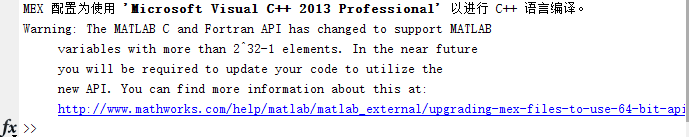第三步、之后就是进行对MATLAB中的function的编译了。这里，我的函数名为phasecong，实现的是图像相位一致性的计算。如下图所示。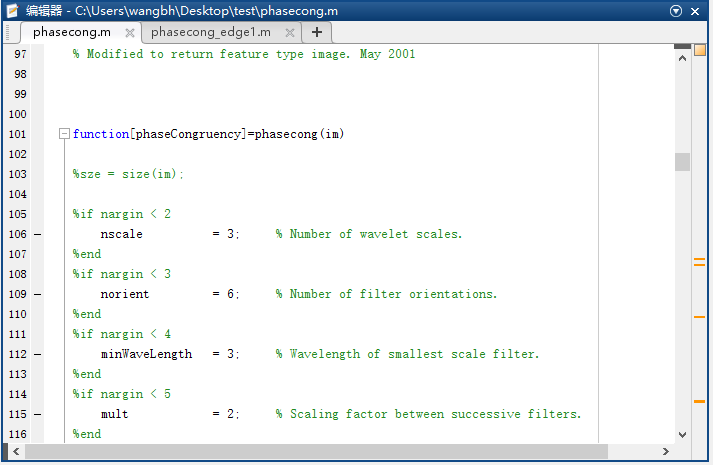这个函数是一位大牛学者写的，原本是有多个输入和多个输出的，但是为了编译方便和后续调用方便，我把其他几个参数删掉了，只保留了一个输入矩阵和一个输出矩阵。

这样做的好处是可以减少编译和调用出错的概率。编译方法见第四步。

第四步、下面就是对function进行编译了，编译的命令为：mcc -W cpplib:pc -T link:lib phasecong

这条命令中pc表示的是想要生成的库的名称，可以自己定，最后面的phasecong表示的是要编译的函数的名，注意函数名后面不要加.m后缀。其他的参数都不用改。

如下图所示。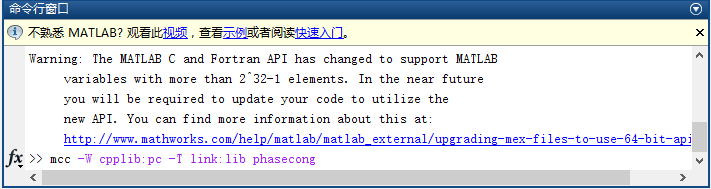第五步、输入完上面的命令后就是等待MATLAB编译完成了，我当时编译好像用了30秒左右的时间。

编译完成后就会出现编译成功的提示，如下图所示。需要注意的是，在编译时，当前文件夹一定要为function所在的文件夹。

第六步、经过上面的编译后，在该文件夹下就会生成.h .cpp .lib .dll这四个文件，因为我设置的输出的库名称为pc，因此我这里生成pc.h pc.cpp pc.lib pc.dll这四个文件。第七步、接下来就是将生成的这四个文件加入到我们的C++工程中了。

将这四个文件放在C++工程的文件夹中，最好在该文件夹中新建两个文件夹，分别命名为lib和include，因为后面我们还需要引入其他的库文件和头文件，这样做可以让文件更整齐。然后将pc.lib放入lib文件夹，将pc.h放入include文件夹。然后在VS中右键项目，点击属性，在属性中选择“配置属性”->“VC++目录”->“包含目录”，将刚才建立的include文件夹包含进去，如下图所示。其他的属性都不用改。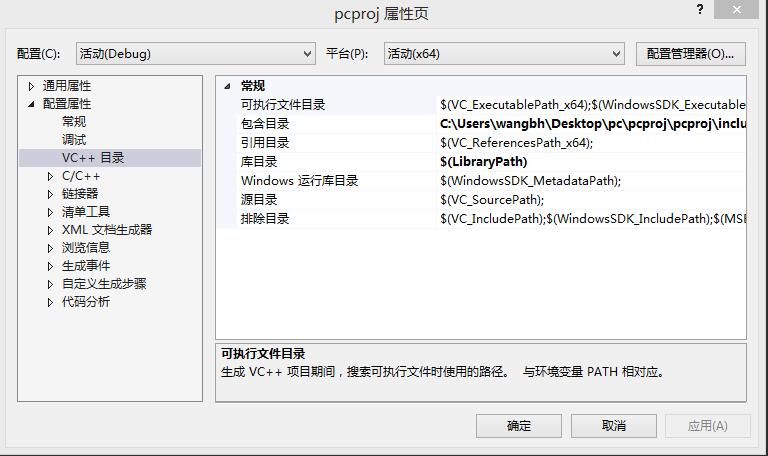这样我们就将头文件包含了进去，之后进行加载lib文件，这里我们使用静态加载方式，在工程文件中加入这样一条语句：

#pragma comment(lib, ".\\lib\\pc.lib" )

对于pc.dll和pc.cpp文件，值需要将其放在工程目录下就可以，不用进行配置。
这样就完成了对这四个文件的配置。

第八步、配置好生成的这四个文件后就可以运行C++的程序了，但是点击运行后应该会报错（通常一定会报错），不要怕，这是因为我们前面生成的这四个文件是需要依赖其他的库和头文件才能工作的。我们根据报错的信息可以知道缺少的头文件，例如下图这个错误：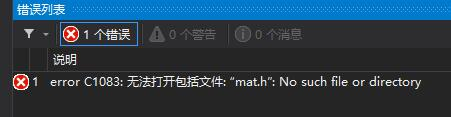通过这个错误我们就可以知道，我们的项目中缺少mat.h这个头文件，这时我们就要找到这个头文件并将其加入到include文件夹中。到哪里去找呢，在这个文件夹中“D:\Program Files\MATLAB\R2014b\extern\include”。重复这一操作，直到不再提示这种错误。这一操作需要重复很多次，像我这个项目需要加入下图这么多的头文件。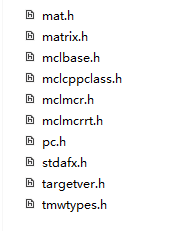之后还需要加入额外的lib库文件，具体加入哪些lib文件，需要根据上面的这些头文件而定，我们到文件夹“D:\Program Files\MATLAB\R2014b\extern\lib\win64\microsoft”中（因为我的MATLAB是64位的，所以在win64文件夹下，如果大家的MATLAB是32位的，那么估计是在win32文件夹下，大家自己找一下），寻找上面这些头文件同名的lib文件，不过并不是每个头文件都能找到同名的lib文件，不过不要紧，我们只要把能找到的lib文件复制到工程目录下的lib文件夹中即可，除了这些lib文件之外，还需要加上“libmat.lib”和“libmx.lib”这两个文件，这两个文件好像包括着必要的类型的定义之类的吧。搞定之后我的lib文件夹中有下图这些个文件。

这些lib的加载方式与之前我们说的pc.lib的加载方式相同，如下图所示。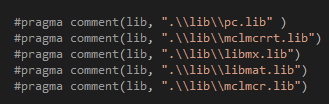进行到此，我们所有的配置就都搞定了，如果没有意外的话，C++的项目编译就可以通过了，

但是虽然编译通过了，在运行的过程中还是有可能提示缺少某个库文件，我们只需要采用同样的方法把那些库文件加到项目里即可，比如我这个程序还提示我缺少mclmcr.dll这个文件，我就把这个文件复制到工程目录下就可以了，所需的dll文件通常都在“D:\Program Files\MATLAB\R2014b\bin\win64”这个文件夹可以找到。

至此我们就完成了MATLAB函数的编译和C++调用的整个过程。

最后是几点提示：

1、我这里使用的是MATLAB2014a和vs2013。

2、如果使用的MATLAB是64位的版本，那么C++的程序也要在x64下配置！！！！！！！

如果使用的MATLAB是32位的版本，那么C++的程序也要在win32下配置！！！！！！

这点非常重要！！！！！！！！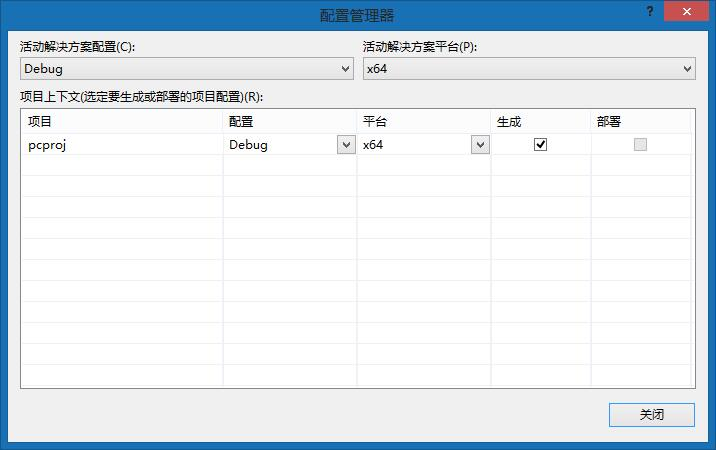3、MATLAB函数的输入和输出参数通常都是矩阵，在C++中矩阵变量使用mwArray这个类型，这个类的使用方法可以看我下面贴的代码，也可以在网上找教程。

4、在C++程序中，我们调用这个函数的名称与MATLAB中定义的函数名称相同，这个函数是在MATLAB编译后生成的头文件中进行的声明，例如我这里是在pc.h中。

5、在C++程序中，在定义输入数组和输出数组时，最好使用动态数组，因为传统的数组是在栈中分配内存，这样会造成内存溢出。

最后为了更直观的展示在C++中如何对这些个库文件进行的调用以及如何使用这个函数，我在这里贴上了C++程序的源代码。

// pcproj.cpp : 定义控制台应用程序的入口点。
//

#include "stdafx.h"
#include "pc.h"
#include <iostream>
#include <fstream>

using namespace std;

#pragma comment(lib, ".\\lib\\pc.lib" )
#pragma comment(lib, ".\\lib\\mclmcrrt.lib")
#pragma comment(lib, ".\\lib\\libmx.lib")
#pragma comment(lib, ".\\lib\\libmat.lib")
#pragma comment(lib, ".\\lib\\mclmcr.lib")

#define WIDTH 512
#define HEIGHT 512

int _tmain(int argc, _TCHAR* argv[])
{
//mclInitializeApplication(NULL, 0);
//mclmcrInitialize();

UINT8 *img_befor = new UINT8[WIDTH*HEIGHT];
DOUBLE *img_after = new DOUBLE[WIDTH*HEIGHT];

int data;
double ddata;
FILE *fp;

errno_t err = fopen_s(&fp, "pixel.txt", "r");
if (err!=0){
cout << "open pic fail!" << endl;
return 0;
}

for (int i = 0; i < WIDTH; i++){
for (int j = 0; j < HEIGHT; j++){
fscanf_s(fp, "%d", &data);
img_befor[i*WIDTH + j] = data;
}
}

fclose(fp);
fp = NULL;

pcInitialize();

mwArray img_input_array(HEIGHT, WIDTH, mxUINT8_CLASS, mxREAL);
mwArray img_output_array(HEIGHT, WIDTH, mxDOUBLE_CLASS, mxREAL);
int nargout = 1;

img_input_array.SetData(img_befor, HEIGHT*WIDTH);

phasecong(nargout,img_output_array,img_input_array);

img_output_array.GetData(img_after, HEIGHT*WIDTH);

pcTerminate();

//将结果写到文件
err = fopen_s(&fp, "pixel_after.txt", "w");
if (err != 0){
cout << "open pic_after fail!" << endl;
}
else{
//写文件
for (int i = 0; i < WIDTH; i++){
for (int j = 0; j < HEIGHT; j++){
ddata = img_after[i*WIDTH + j];
fprintf(fp, "%.4f ", ddata);
}
fprintf(fp, "\n");
}
}
fclose(fp);
fp = NULL;

return 0;
}展开全文• c++调用matlab函数，踩了不少坑，终于搞定

环境：matlab2018a   ； visual studio2017

一、写好matlab函数，这里设为resCode.m

二、接下来生成c++所需文件

步骤1：mex -setup

点击选择mex -setup  C++

步骤2：mbuild -setup

点击选择mex -setup  C++ -client MBUILD

步骤3：mcc -W cpplib: resCode -T link:lib resCode.m -C

resCode是你的dll名称，可以自定义，resCode.m就是你的m文件名称

解释：

-W是控制编译之后的封装格式；

cpplib是指编译成C++的lib，如果需要编译成C的lib，就用lib即可，去掉cpp；

cpplib后面的是需要生成文件的文件名，是自己取的，我们取名为resCode；

其他具体含义可以通过mcc –help命令查看，注意参数的大小写。

需要用到的文件只有四个：resCode.ctf，resCode.dll，resCode.lib，resCode.h。

三、C++配置部分

创建工程文件，注意：测试环境使用的是64位操作系统，因此务必在x64环境下进行以下配置。（这是与你的matlab版本匹配的，我的matlab安装的是64bit的）

第一步：VC++目录

假设Matlab安装目录为D:\Matlab2018a，这个根据本机安装目录自行调整。

包含目录：D:\Matlab2018a\extern\include

D:\MATLAB 2018a\extern\include\win64
D:\project\IMU_PointCloud_predict\workspace  //工程的工作目录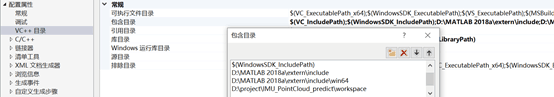库目录：D:\Matlab2018a\extern\lib\win64\microsoft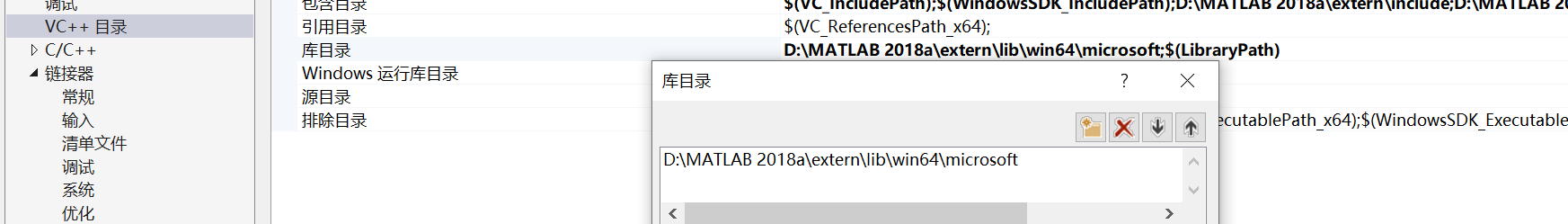第二步：链接器->输入->附加依赖项，添加以下库

mclmcrrt.lib

libmat.lib

libmx.lib

mclmcr.lib

resCode.lib //自己生成的lib文件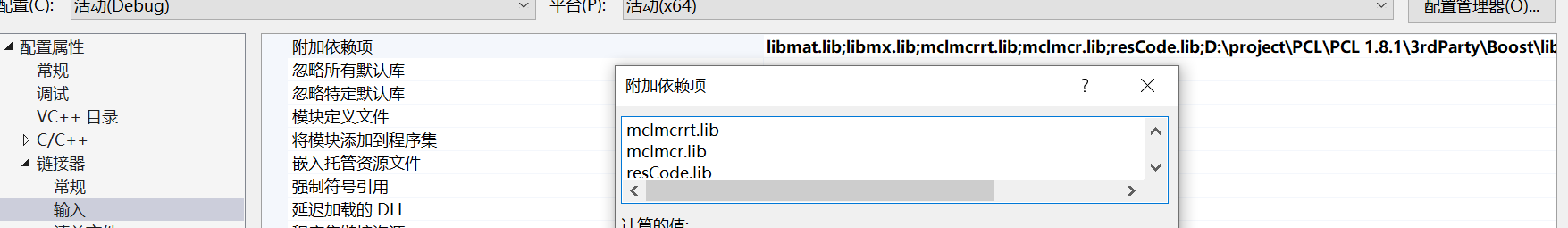第三步：配置系统变量

“我的电脑”右键，打开“属性”；选择“高级系统设置”；找到系统属性页面的“高级”选项，右下方“环境变量”；页面中下方是系统变量，将Path设置为D:\Matlab2018a\bin\win64

进行完以上配置后，将电脑进行重启（让环境变量生效）。

第四步：重新打开VS2017的项目，将Matlab生成的四个文件放入该项目所在文件夹下。（注意：我看了网上很多教程，放在哪里哪里，但是最终试了一下，只需要把四个文件放在工程底下即可，上面配置配置完成即可，这一步非常重要）。

第五步：调试–>窗口–>异常设置–>Win32 Exceptions全部不要勾选。

至此完成VS的配置

四、测试部分（这里展示字符串如何作为参数输入到matlab函数）

这是我写的matlab函数，输入参数为字符串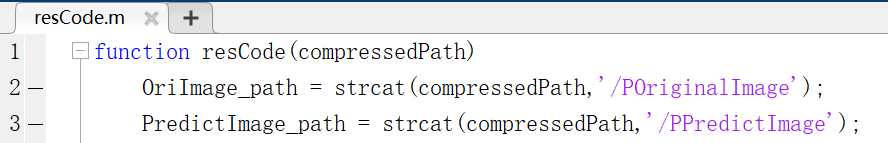这是c++调用matlab程序：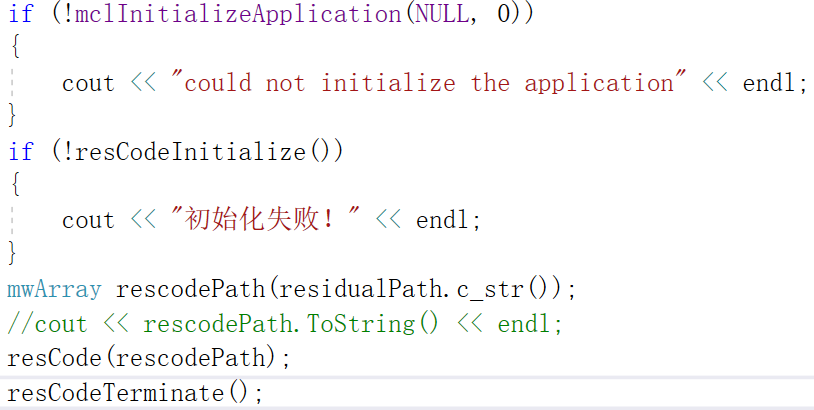有几点注意点：一定要初始化两句

mclInitializeApplication(NULL, 0)

resCodeInitialize()  //resCode是你的函数名字

其次，不管想把矩阵或者字符串作为参数输入到Matlab中，都要变成mwArray格式的参数，才能调用函数输入参数。这里展示字符串作为参数。

说明：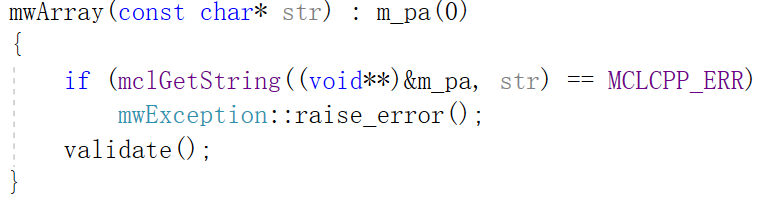residualPath是string类型，上面是mwArray类形式的一部分，想看全部可以去看内部函数。它可以把const char* str作为参数将字符串变成mwArray，又因为string a.c_str()返回的类型也是const char*类型，因此可以将字符串转成mwArray类型，然后才能当作参数输入到matlab函数。最后加上结束调用resCodeTerminate()。

展开全文• c++调用Matlab函数画图，示例代码，具体过程参照我的博客中的两篇文章
• Visual C++调用MATLAB函数库的混合编程技术.pdf
• Visual C++调用MATLAB函数库的混合编程技术
• 本文件是C++调用MATLAB的程序，平台是VS2012和MATLAB 2017，这里的平台不能随意更换，因为存在兼容性问题。程序整体已经通过测试。相关的混合编程理论可见我的博客文章。
• C++与matlab混合编程——C++调用MATLAB函数 笔者最近在从事一个MFC相关的项目，要求将用Matlab实现的算法通过应用MFC制作成一个小应用。其中有一部分内容需要求一个多元函数的最值。通过网络，我找到了两个C++优化...
• 想用C++调用matlab函数，完全按照网上的教程走的，但是运行后没有结果出来，调试时发现调用那一步说什么mcr.pdb找不到，在安装的mcr文件夹下搜也没找到这个文件。有没有好心人知道怎么处理这种情况能够指导一下，...
• 项目中遇到一个问题，需要用c++调用matlab程序，这里记录下整个过程。 准备环境 matlab 2014a visual studio 2010 PS：版本问题要注意，之前是visual studio 2017，这个就一直报错（笑cry），matlab比较难安装...
• 主要介绍了C++如何调用matlab函数的相关资料,非常不错，具有参考借鉴价值，需要的朋友可以参考下
• 此篇紧接上篇实现c++调用MATLAB函数。 Ubuntu下用c++调用自己编写的matlab函数编写matlab函数生成动态链接库阅读readme.txt文件Prerequisites for Deployment（部署的前提条件）1. enter2. run the MATLAB Runtime ...linux
• 从vs2015中调用matlab中自定义的函数c++和matlab的混合编程简单实例
• C++调用Matlab主要有两种方式，一种是调用Matlab的计算引擎，即调用Matlab本身；另一种是调用matlab中由mcc编译生成的dll文件，这种方法是Matlab利用C++ Complier将m文件转化为C++源码，产生能够完全脱离Matlab运行...
• ## C/C++ VS中调用matlab函数的方法

万次阅读 多人点赞 2017-07-01 16:20:01
C/C++ VS中调用matlab函数的方法 Matlab在数值等矩阵运算中具有很大优势，并且Matlab提供了强大的工具箱可以使用，在C/C++开发中，我们可以在VS下调用matlab函数进行混合编程。下面主要介绍VS中调用matlab函数的环境...
• 本文将尝试另外一种途径，通过matlab引擎来实现c++调用matlab中自己编写的m函数。既然之前已经配置好matlab引擎，配置完以后就可以通过引擎提供的函数engEvalString，向matlab发送命令。直接调用matlab自带的函数...
• 学习c++与matlab混合编程一般是通过c++调用matlab函数，因为matlab具有强大的数学函数库，然而vc++具有界面设计灵活的优点，下面这篇文章主要给大家介绍了关于在ubuntu系统下C++调用matlab程序的方法，需要的朋友...
• 在项目中，遇到需要用c++调用matlab程序，这里记录下整个过程。以供遇到类似问题的人作参考。此问题棘手的原因主要有在MATLAB编写的项目程序中用到了很多深度学习库（FLANN、vlfeat库）和caffe框架，经过多次尝试，...深度学习...

# c++调用matlab函数c++ 订阅matlab 订阅